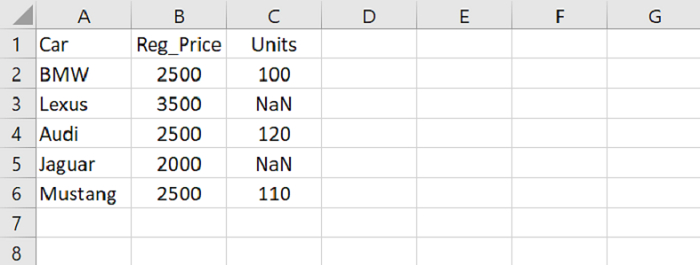# Python Pandas – Propagate non-null values forward

PythonServer Side ProgrammingProgramming

Use the “method” parameter of the fillna() method. For forward fill, use the value ‘ffill’ as shown below −

fillna(method='ffill')

Let’s say the following is our CSV file opened in Microsoft Excel with some NaN values −At first, import the required library −

import pandas as pd


Load data from a CSV file into a Pandas DataFrame −

dataFrame = pd.read_csv("C:\\Users\\amit_\\Desktop\\SalesData.csv")

## Example

Following is the complete code −

import pandas as pd

# Load data from a CSV file into a Pandas DataFrame
print("DataFrame...\n",dataFrame)

# propagate non null values forward
res = dataFrame.fillna(method='ffill')
print("\nDataFrame after forward fill...\n",res)

## Output

This will produce the following output −

DataFrame...
Car   Reg_Price   Units
0      BMW        2500   100.0
1    Lexus        3500     NaN
2     Audi        2500   120.0
3   Jaguar        2000     NaN
4  Mustang        2500   110.0

DataFrame after forward fill...
Car   Reg_Price   Units
0      BMW        2500   100.0
1    Lexus        3500   100.0
2     Audi        2500   120.0
3   Jaguar        2000   120.0
4  Mustang        2500   110.0